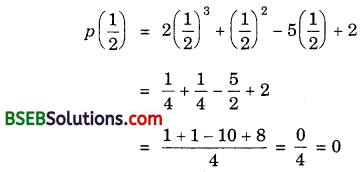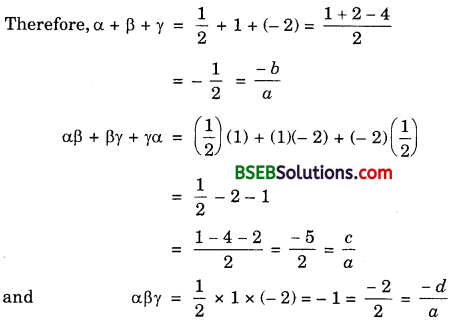# Bihar Board Class 10th Maths Solutions Chapter 2 Polynomials Ex 2.4

Bihar Board Class 10th Maths Solutions Chapter 2 Polynomials Ex 2.4 Textbook Questions and Answers.

## BSEB Bihar Board Class 10th Maths Solutions Chapter 2 Polynomials Ex 2.4

Question 1.
Verify that the numbers given alongside of the cubic polynomials below are their zeros.
Also verify the relationship between the zeroes and the coefficients in each case:

1. 2x3 + x2 – 5x + 2; $$\frac{1}{2}$$, 1, – 2
2. x3 – 4x2 + 5x – 2; 2, 1, 1

Solution:
1. Comparing the given polynomial with ax3 + bx2 + cx + d, we get
a = 2, b = 1, c = – 5 and d = 2.p(1) = 2(1)3 + (1)2 – 5(1) + 2
= 2 + 1 – 5 + 2 = 0
p(- 2) = 2(- 2)3 + (- 2)2 – 5(- 2) + 2
= 2(- 8) + 4 + 10 + 2
= – 16 + 16 = 0
∴ $$\frac{1}{2}$$, 1 and – 2 are the zeroes of 2x3 + x2 – 5x + 2.
So, α = $$\frac{1}{2}$$, β = 1 and γ = – 2.2. Comparing the given polynomial with ax3 + bx2 + cx + d, we get
a = 1, b = – 4, c = 5 and d = – 2.
p(2) = (2)3 – 4(2)2 + 5(2) – 2
= 8 – 16 + 10 – 2 = 0
p(1) = (1)3 – 4(1)2 + 5(1) – 2
= 1 – 4 + 5 – 2 = 0
∴ 2, 1 and 1 are the zeroes of x3 – 4x2 + 5x – 2.
So, α = 2, β = 1 and γ = 1.
Therefore, α + β + γ = 2 + 1 + 1 = 4 = $$\frac{-(-4)}{1}$$ = $$\frac{-b}{a}$$
αβ + βγ + γα = (2)(1) + (1)(1) + (1)(2)
= 2 + 1 + 2 + 5 = $$\frac{5}{1}$$ = $$\frac{c}{a}$$
and αβγ = (2)(1)(1) = 2 = $$\frac{-(-2)}{1}$$ = $$\frac{-d}{a}$$.Question 2.
Find a cubic polynomial with the sum, sum of the products of its zeroes taken two at a time, and the product of its zeroes as 2, – 7, – 14 respectively.
Solution:
Let the cubic polynomial be ax3 + bx2 + cx + d, and its zeroes be α, β and γ.
Then, α + β + γ = 2 = $$\frac{-(-2)}{1}$$ = $$\frac{-b}{a}$$
αβ + βγ + γα = – 7 = $$\frac{-7}{1}$$ = $$\frac{c}{a}$$
and αβγ = – 14 = $$\frac{-14}{1}$$ = $$\frac{-d}{a}$$
If a = 1, then b = – 2, c = – 7 and d = 14.
So, one cubic polynomial which fits the given conditions x3 – 2x2 – 7x + 14.

Question 3.
If the zeroes of the polynomial x3 – 3x2 + x + 1 are a – b, a and a + b, find a and b.
Solution:
Since (a – b), a and (a + b) are the zeroes of the polynomial x3 – 3x2 + x + 1, therefore
So, (a – b) + a + (a + b) = $$\frac{-(-3)}{1}$$ = 3
So, 3a = 3 or a = 1
(a – b)a + a(a + b) + (a + b)(a – b) = $$\frac{1}{1}$$ = 1
or a2 – ab + a2 + ab + a2 – b2 = 1
or 3a2 – b2 = 1
So, 3(1)2 – b2 = 1 [∵ a = 1]
or 3 – b2 = 1
or b2 = 2 or b = ± $$\sqrt{2}$$
Hence, a = 1 and b = ± $$\sqrt{2}$$.Question 4.
If two zeroes of the polynomial x4 – 6x3 – 26x2 + 138x – 35 are 2 ± $$\sqrt{3}$$, find other zeroes.
Solution:
We have:
2 ± $$\sqrt{3}$$ are two zeroes of the polynomial p(x) = x4 – 6x3 – 26x2 + 138x – 35
Let x = 2 ± $$\sqrt{3}$$. So, x – 2 = ± $$\sqrt{3}$$
Squaring, we get
x2 – 4x + 4 = 3, i.e; x2 – 4x + 1 = 0
Let us divide p(x) by x2 – 4x + 1 to obtain other zeroes.∴ p(x) = x4 – 6x3 – 26x2 + 138x – 35
= (x2 – 4x + 1)(x2 – 2x – 35)
= (x2 – 4x + 1)(x2 – 7x + 5x – 35)
= (x2 – 4x + 1)[x(x – 7) + 5(x – 7)]
= (x2 – 4x + 1)(x + 5)(x – 7)
So, (x + 5) and (x – 7) are other factors of p(x).
∴ – 5 and 7 are other zeroes of the given polynomial.Question 5.
If the polynomial x4 – 6x3 + 16x2 – 25x + 10 is divided by another polynomial x2 – 2x + k, the remainder comes out to be x + a, find k and a.
Solution:
Let us divide x4 – 6x3 + 16x2 – 25x + 10 by x2 – 2x + k.∴ Remainder = (2k – 9)x – (8 – k)k + 10
But the remainder is given as x + a.
On comparing their coefficients, we have:
2k – 9 = 1 or 2k = 10 or k = 5
and – (8 – k)k + 10 = a
So, a = – (8 – 5)5 + 10
= – 3 × 5 + 10 = – 15 + 10 = – 5
Hence, k = 5 and a = – 5.